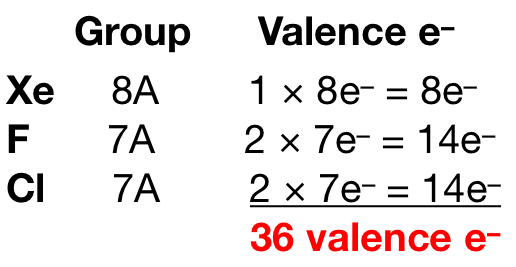# Problem: Two different compounds have the formula XeF2Cl2. Write Lewis structures for these two compounds, and describe how measurement of dipole moments might be used to distinguish between them.

###### FREE Expert Solution

Xenon (EN = 2.60) is less electronegative than fluorine (EN = 3.98) and chlorine (EN = 3.16) so xenon is the central atom. We then need to count the total number of valence electrons for each element:Fluorine and chlorine prefers to form 1 bond. There are two possible Lewis structures for this compound:

79% (437 ratings)###### Problem Details

Two different compounds have the formula XeF2Cl2. Write Lewis structures for these two compounds, and describe how measurement of dipole moments might be used to distinguish between them.